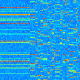## About Calvin McCarter

PhD Student School of Computer Science Carnegie Mellon University

# Resizing nested C++ STL vectors

“Multidimensional” vectors in C++ do not behave like matrices (or higher-order equivalents). Something like:

vector< vector<double> > foo(100, vector<double>(20, 0.0));

will not lay out 100*20 doubles contiguously in memory. Only the bookkeeping info for 100 vector<double>’s will be laid out contiguously — each vector<double> will store its actual data in its own location on the heap. Thus, each vector<double> can have its own size.

This can lead to hard-to-catch bugs:

 foo.resize(300, vector<double>(30, 1.0));

will leave the first 100 vector<double>’s with size 20, filled with 0.0 values, while the new 200 vector<double>’s will have size 30, filled with 1.0 values.

# Sampling from Multivariate Gaussian distribution in Matlab

tl;dr: Don’t use mvnrnd in Matlab for large problems; do it manually instead.

The first improvement uses the Cholesky decomposition, allowing us to sample from a univariate normal distribution. The second improvement uses the Cholesky decomposition of the sparse inverse covariance matrix, not the dense covariance matrix. The third improvement avoids computing the inverse, instead solving a (sparse) system of equations.

 n = 10000; Lambda = gallery('tridiag',n,-0.3,1,-0.3); % sparse tic; x_mvnrnd = mvnrnd(zeros(n,1),inv(Lambda)); toc;

 tic; z = randn(n,1); % univariate random Sigma = inv(Lambda); A = chol(Sigma,'lower'); % sparse x_fromSigma = A*z; % using cholesky of Sigma toc; tic; z = randn(n,1); % univariate random L_Lambda = chol(Lambda,'lower'); % sparse A_fromLambda = (inv(L_Lambda))'; % sparse x_fromLambda = A_fromLambda*z; toc; 

tic; z = randn(n,1); % univariate random L_Lambda = chol(Lambda,'lower'); % sparse x_fromLambda = L_Lambda'\z; toc; 

Results:
 Elapsed time is 4.514641 seconds. Elapsed time is 2.734001 seconds. Elapsed time is 1.740317 seconds. Elapsed time is 0.012431 seconds. 

# Matlab: different colormaps for subplots

I often want different subplots in one Matlab figure to have different colormaps. However, colormap is a figure property, so it’s not trivial, except that it is… with these utilities:

This works for everything except colorbars: http://www.mathworks.com/matlabcentral/fileexchange/7943-freezecolors—unfreezecolors

Post-2010, Matlab refreshes colorbars with each subplot, so you’ll need this to freeze colorbars: http://www.mathworks.com/matlabcentral/fileexchange/24371-colormap-and-colorbar-utilities–feb-2014-

# Scaling up hierarchical clustering

There are lots of caveats with hierarchical clustering, and it’s often used to draw unjustifiable conclusions. But I’ll save that discussion for later, and it’s at least occasionally useful. 🙂 So far, I’ve mainly used it to reorder the columns/rows of a covariance or correlation matrix. For example, I recently generated synthetic sparse precision matrices, and I wanted to make sure that the corresponding covariance/correlation matrices were as I expected.

However, linkage/dendrogram in both Matlab and SciPy are really slow. In particular, the linkage algorithms they use are O(n^3). So instead we can use fastcluster, which is O(n^2). All I had to do was replace this:

Z = sch.linkage(P, method="average", metric="correlation")

with this:

Z = fastcluster.linkage(P, method="average", metric="correlation")

The second problem is that dendrogram does lots of unnecessary work when all I want is the reordered indices. SciPy actually implements it recursively, so it gives this error: “RuntimeError: maximum recursion depth exceeded”. So the second change is to replace this:

dendr = sch.dendrogram(Z,no_plot=True,distance_sort=True)ix = dendr["leaves"]

with this (credit to a good denizen of StackOverflow):

n = len(Z) + 1cache = dict()
for k in range(len(Z)):    c1, c2 = int(Z[k]), int(Z[k])    c1 = [c1] if c1 < n else cache.pop(c1)    c2 = [c2] if c2 < n else cache.pop(c2)    cache[n+k] = c1 + c2ix = cache[2*len(Z)]

Then it’s as simple as:

Pclust = P[ix,:]Pclust = Pclust[:,ix]pyplot.imshow(Pclust, interpolation="nearest")

“Three unblinded mice” from Andrew Gelman’s blog

I really like the phrase “pre-scientific intuitions”. Also this: “OK, sure, sure, everybody knows about statistics and p-values and all that, but my impression is that researchers see these methods as a way to prove that an effect is real. That is, statistics is seen, not as a way to model variation, but as a way to remove uncertainty.”

# Converting between decimal and unary in Matlab

Decimal to unary:

[1:4]'==3   % returns [0 0 1 0 ]

Unary to decimal:

max([0 0 1 0] .* (1:4))   % returns 3

# Fisher information, score function, oh my!

Ever wondered why$\text{Var}_{\theta}(\ell'(\theta)) = I_n(\theta)$ and$\text{Var}_{\theta}(\hat{\theta}) \approx \frac{1}{I_n(\theta)}$?

This is an excellent intuitive explanation:

http://math.stackexchange.com/a/265933/105416

.

# Confidence Intervals from Pivots

This is an example of using a pivot to find a confidence interval.$X_1,...,X_n \sim \text{Uniform}(0,\theta).$

1. Find a pivot:

Let$Q=X_{(n)}/\theta$.

2. Find its distribution:$P(Q \le t)= P(X_i \le t\theta)^n = t^n$.

3. Find an expression involving an upper and lower bound on the pivot:$P(a \le Q \le b) = b^n-a^n$ This implies$P(a \le Q \le 1) = 1-a^n$.

4. Substitute the expression for the pivot from Step 1, and set the RHS to$1-\alpha$.$P(a \le X_{(n)}/\theta \le 1)=1-a^n$$P(1/a \ge \theta/X_{(n)} \ge 1) = 1-a^n$$P( X_{(n)} \le \theta \le \frac{X_{(n)}}{a} ) = 1-a^n$

Let$1-\alpha = 1-a^n$. Then$a=\alpha^{1/n}$.$P(X_{(n)} \le \theta \le \frac{X_{(n)}}{\alpha^{1/n}})=1-\alpha$

This gives us$[X_{(n)},\frac{X_{(n)}}{\alpha^{1/n}}]$ as a$1-\alpha$ CI for$\theta$.